Ex 3.3

Chapter 3 Class 6 Playing with Numbers
Serial order wise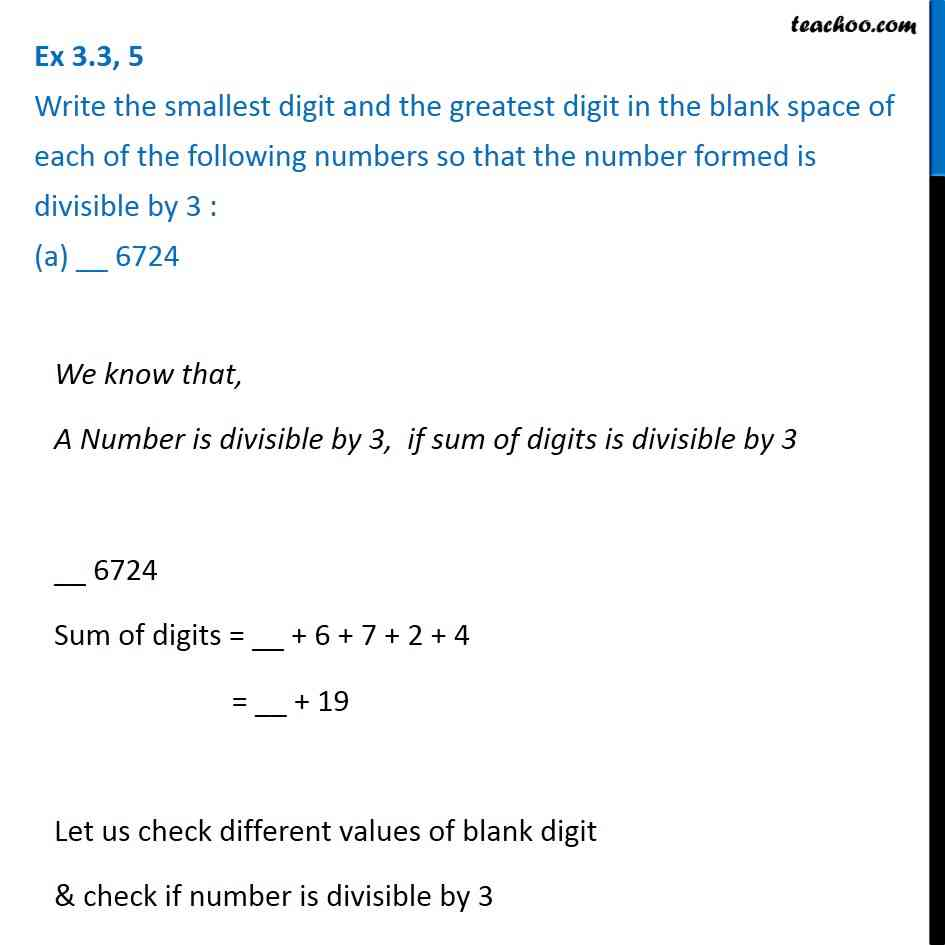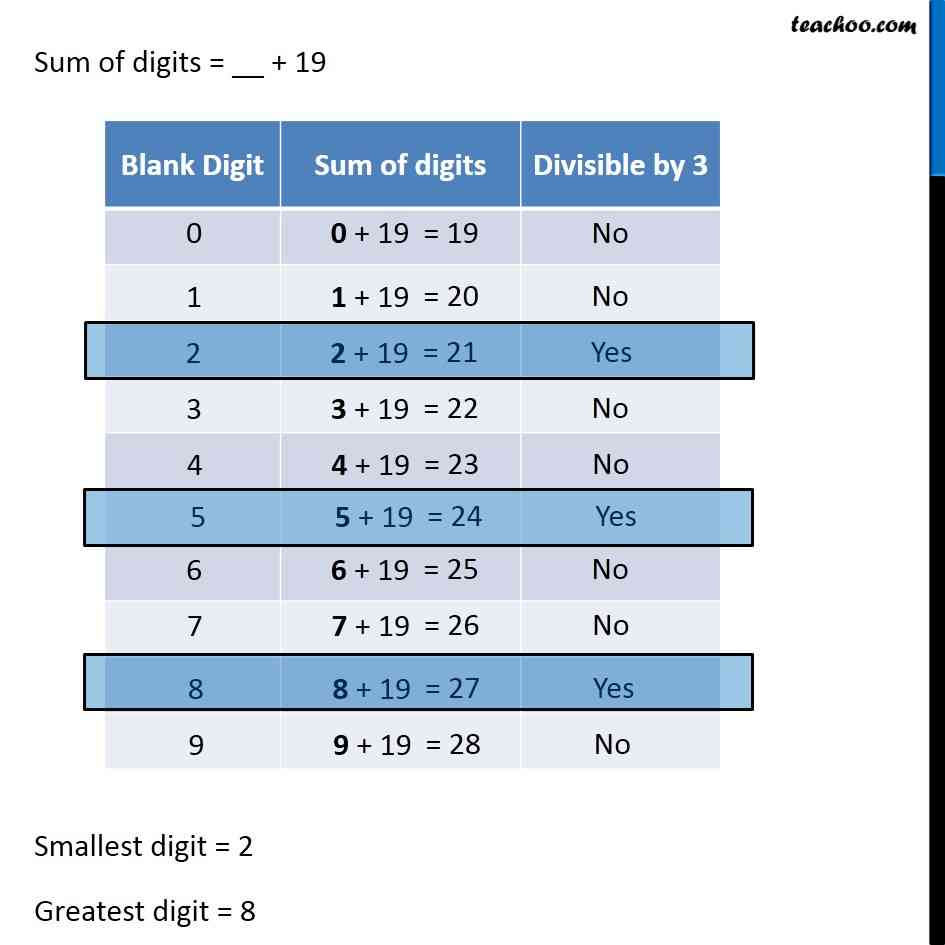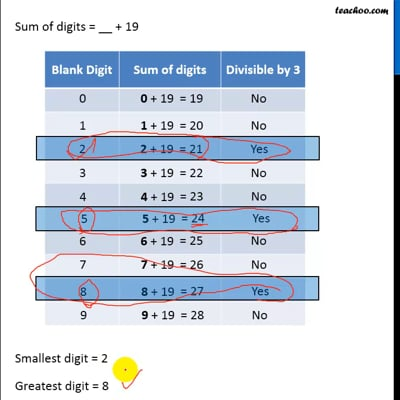This video is only available for Teachoo black users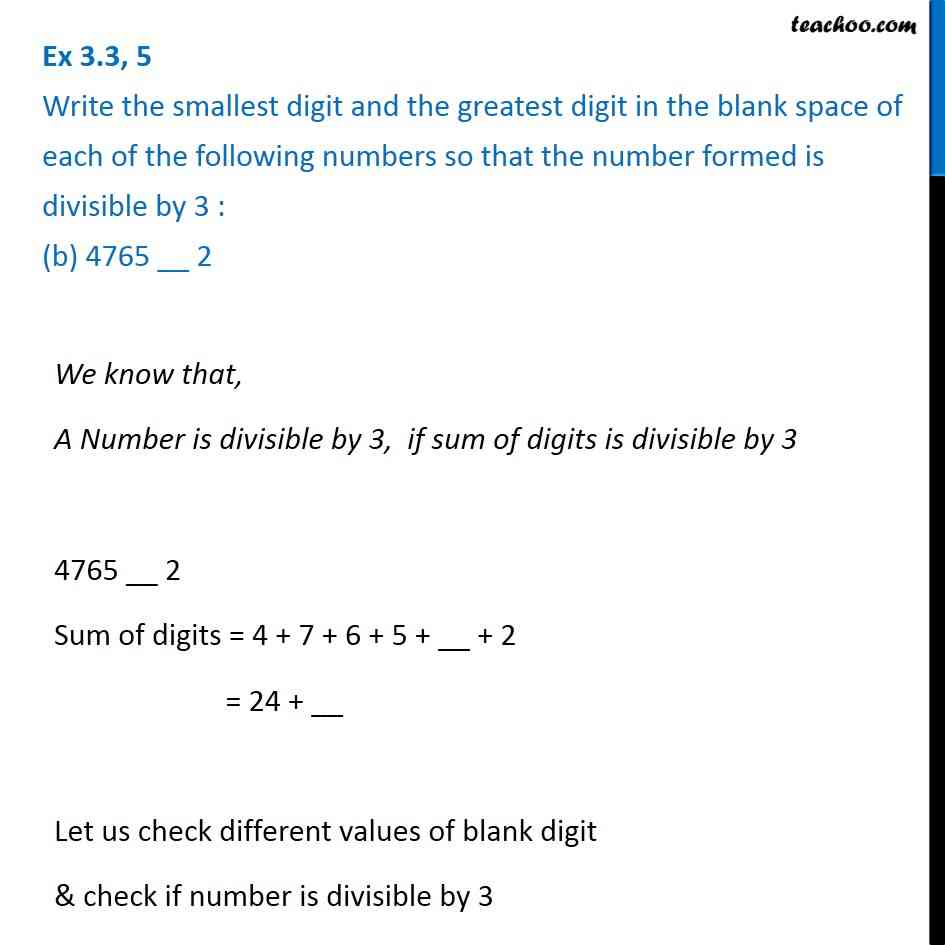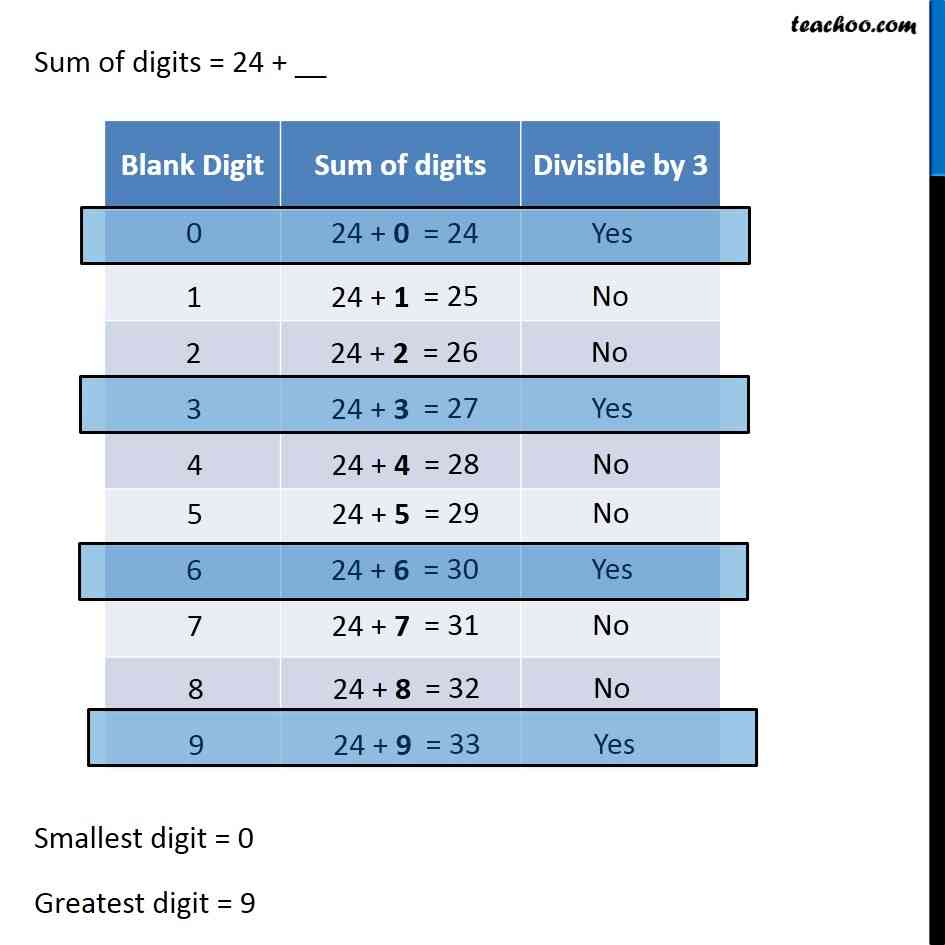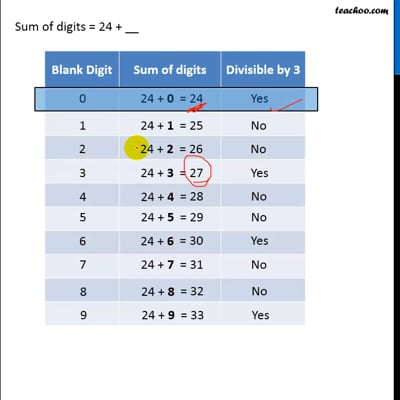This video is only available for Teachoo black users

### Transcript

Ex 3.3, 5 Write the smallest digit and the greatest digit in the blank space of each of the following numbers so that the number formed is divisible by 3 : (a) __ 6724 We know that, A Number is divisible by 3, if sum of digits is divisible by 3 __ 6724 Sum of digits = __ + 6 + 7 + 2 + 4 = __ + 19 Let us check different values of blank digit & check if number is divisible by 3 Sum of digits = __ + 19 Smallest digit = 2 Greatest digit = 8 Ex 3.3, 5 Write the smallest digit and the greatest digit in the blank space of each of the following numbers so that the number formed is divisible by 3 : (b) 4765 __ 2We know that, A Number is divisible by 3, if sum of digits is divisible by 3 4765 __ 2 Sum of digits = 4 + 7 + 6 + 5 + __ + 2 = 24 + __ Let us check different values of blank digit & check if number is divisible by 3 Sum of digits = 24 + __ Smallest digit = 0 Greatest digit = 9